# The boat is traveling along the circular path with a speed of v=(0.0625t2) m/s, where t...

The boat is traveling along the circular path with a speed of v=(0.0625t2) m/s, where t is in seconds. (Figure 1)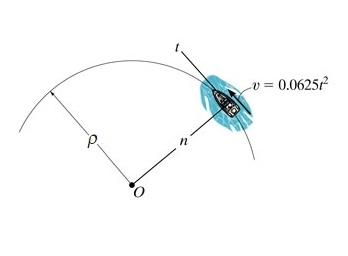Part A

If ? = 42 m , determine the magnitude of its acceleration when t = 12 s .

Express your answer to three significant figures and include the appropriate units.

Concepts and Reason

When an object is moving in a circle, it has two components of acceleration. One component is along the tangent of the circle and the other is towards the center of the circle. They are called tangential and radial/normal acceleration respectively.

The acceleration of the object is the resultant of the radial/normal and tangential accelerations.

Fundamentals

The tangential acceleration follows the laws of motion and is given by,

${a_t} = \frac{{dv}}{{dt}}$

Here, the tangential velocity is v and the time is t.

The normal acceleration is directed towards the center of rotation and is given by,

${a_n} = \frac{{{v^2}}}{R}$

Here, the radius of the circle is R.

Calculate the tangential velocity when $t = 12{\rm{ s}}$.

$\begin{array}{c}\\{\left. v \right|_{t = 12}} = 0.0625{\left( {12} \right)^2}\\\\ = 9{\rm{ m/s}}\\\end{array}$

Calculate the normal acceleration at this instant.

${a_n} = \frac{{{{\left( {{{\left. v \right|}_{t = 12}}} \right)}^2}}}{\rho }$

Here, the radius of the circular path is $\rho$.

Substitute 9 m/s for ${\left. v \right|_{t = 12}}$ and 42 m for $\rho$.

$\begin{array}{c}\\{a_n} = \frac{{{{\left( 9 \right)}^2}}}{{42}}\\\\ = 1.9286{\rm{ m/}}{{\rm{s}}^2}\\\end{array}$

Calculate the tangential acceleration of the boat when $t = 12{\rm{ s}}$.

${a_t} = \frac{{dv}}{{dt}}$

Substitute $0.0625{t^2}$ for v.

$\begin{array}{c}\\{a_t} = \frac{{d\left( {0.0625{t^2}} \right)}}{{dt}}\\\\ = 0.125t\\\end{array}$

$\begin{array}{c}\\{\left. {{a_t}} \right|_{t = 12}} = 0.125 \times 12\\\\ = 1.5{\rm{ m/}}{{\rm{s}}^2}\\\end{array}$

Calculate the total acceleration of the boat.

$a = \sqrt {a_n^2 + a_t^2}$

Substitute $1.9286{\rm{ m/}}{{\rm{s}}^2}$ for ${a_n}$ and $1.5{\rm{ m/}}{{\rm{s}}^2}$ for ${a_t}$.

$\begin{array}{c}\\a = \sqrt {{{1.9286}^2} + {{1.5}^2}} \\\\ = 2.44{\rm{ m/}}{{\rm{s}}^2}\\\end{array}$

Ans:

The magnitude of acceleration of the boat when $t = 12{\rm{ s}}$ is $2.44{\rm{ m/}}{{\rm{s}}^2}$.

#### Earn Coin

Coins can be redeemed for fabulous gifts.

Similar Homework Help Questions
• ### Fundamental Problem 12.19 A particle is traveling along the parabolic path y 0.25c 7 m. U...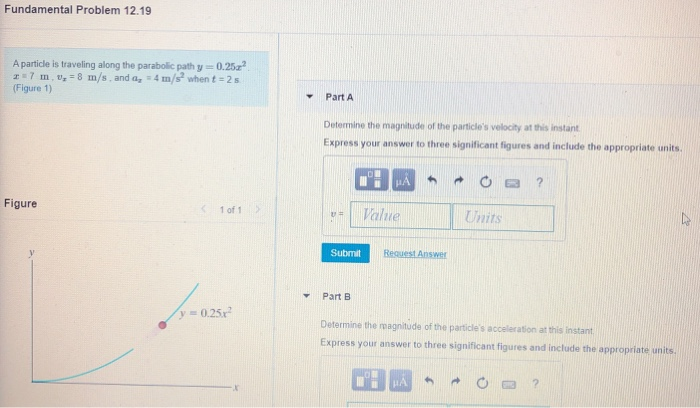Fundamental Problem 12.19 A particle is traveling along the parabolic path y 0.25c 7 m. U = 8 m/s. and ag - 4 m/s when t = 25 (Figure 1) Part A Determine the magnitude of the particle's velocity at this instant Express your answer to three significant figures and include the appropriate units. HAO? ut Value Units Figure 1 of 1 Submit Request Answer Part B y = 0.250 Determine the magnitude of the particles acceleration at this instant...

• ### Part A A particle is traveling along the straight path.(Figure 1) its position along the a...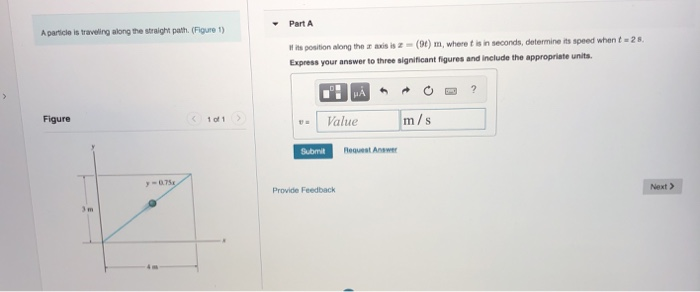Part A A particle is traveling along the straight path.(Figure 1) its position along the a axis is (9t) m, where t is in seconds, determine its speed when t-2s Express your answer to three significant figures and include the appropriste units. Figure 1 of1 U# 1 Value m/s Submit lequest Answer 0.75 Provide Feedback Next >

• ### Problem 12.112 A boat has an initial speed of 12 ft/s . It then increases its...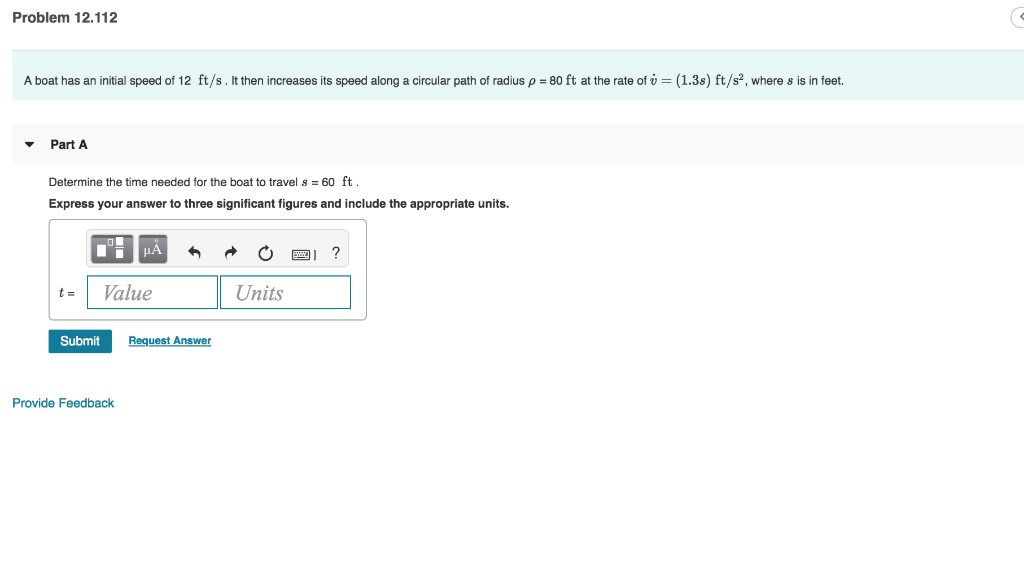Problem 12.112 A boat has an initial speed of 12 ft/s . It then increases its speed along a circular path of radius ρ = 80 ft at the rate of ú = (1.38) ft/s, where s is in feet. Part A Determine the time needed for the boat to travel s = 60 ft Express your answer to three significant figures and include the appropriate units. t= 1 Value Units Submit Request Answer Provide Feedback

• ### Part A The motorcycle is traveling at 50 m/s when it is at A. The speed...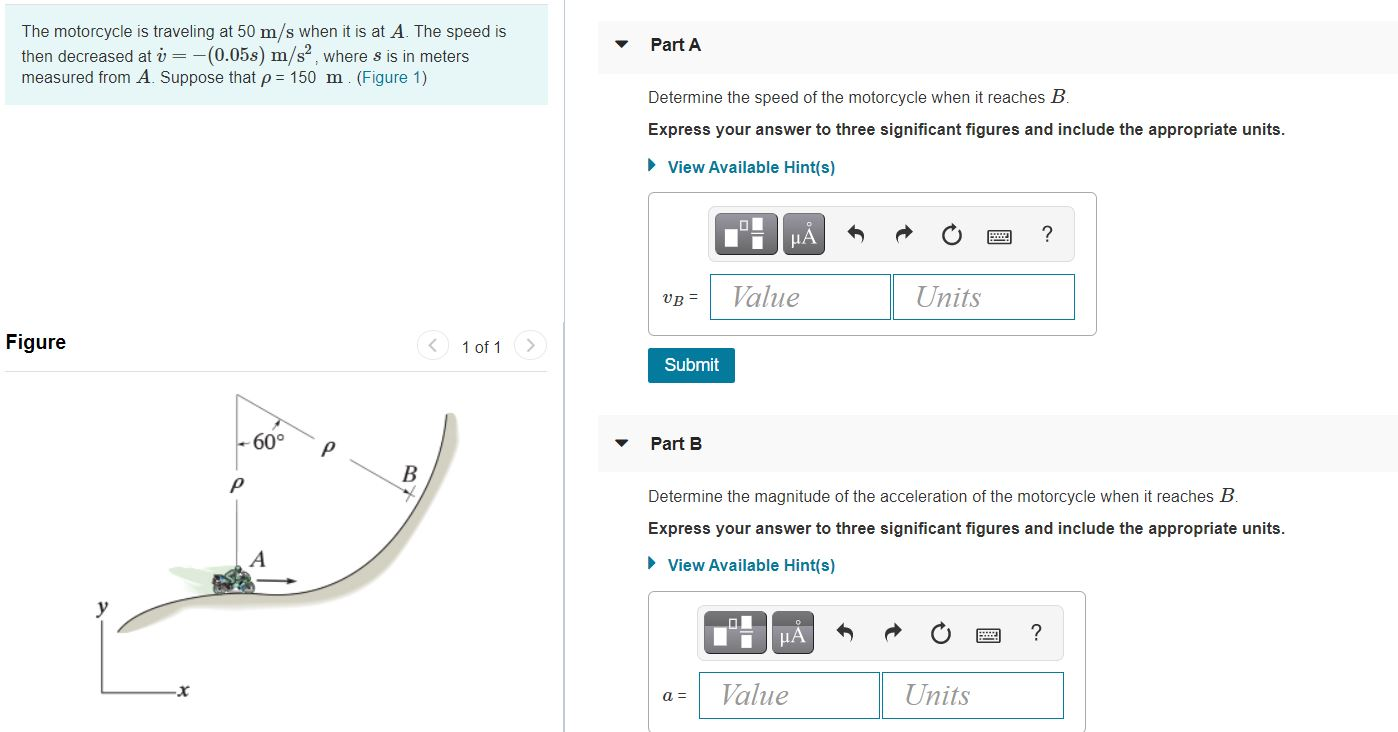Part A The motorcycle is traveling at 50 m/s when it is at A. The speed is then decreased at v= -(0.05s) m/s, where s is in meters measured from A. Suppose that p= 150 m. (Figure 1) Determine the speed of the motorcycle when it reaches B. Express your answer to three significant figures and include the appropriate units. View Available Hint(s) НА ? VB = Value Units Figure < 1 of 1 > Submit -60° р Part B...

• ### Problem 12.112 A boat has an initial speed of 13 ft/s. It then increases its speed...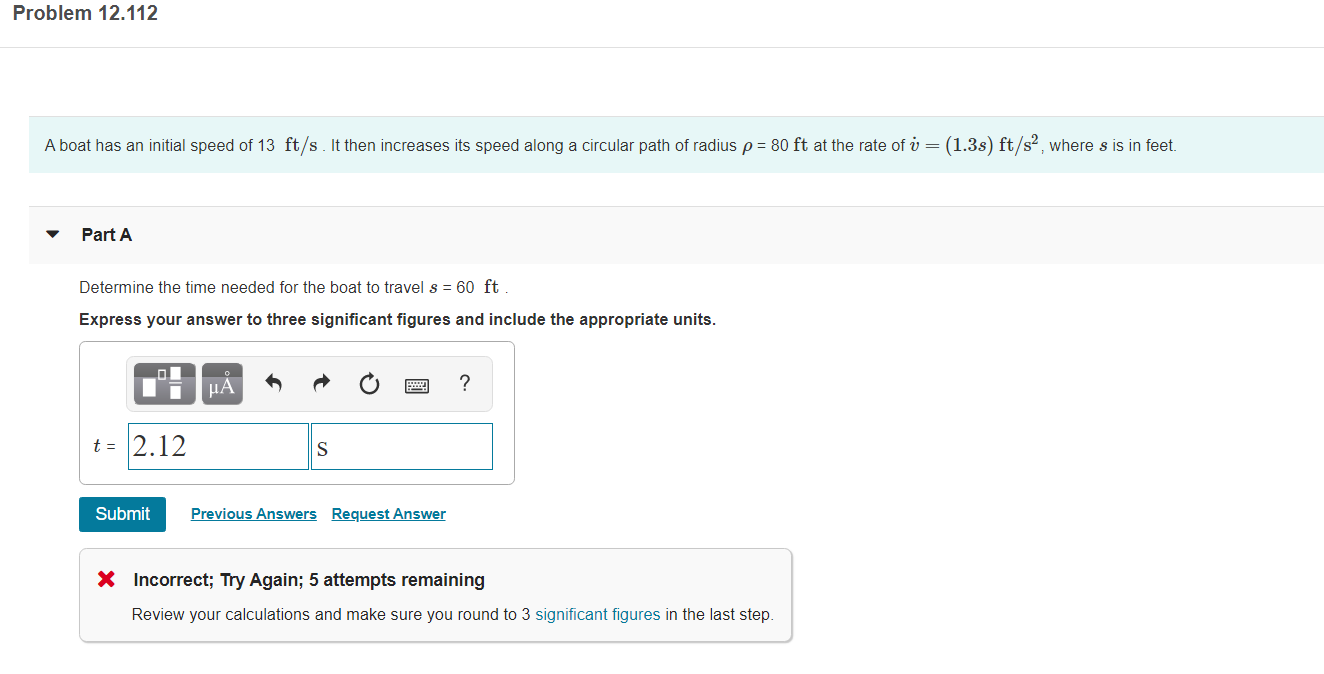Problem 12.112 A boat has an initial speed of 13 ft/s. It then increases its speed along a circular path of radius p = 80 ft at the rate of v = (1.3s) ft/s, where s is in feet. Part A Determine the time needed for the boat to travel s = 60 ft Express your answer to three significant figures and include the appropriate units. t = 2.12 Submit Previous Answers Request Answer X Incorrect; Try Again; 5 attempts...

• ### A particle is moving along a circular path having a radius of 5.4 in. such that...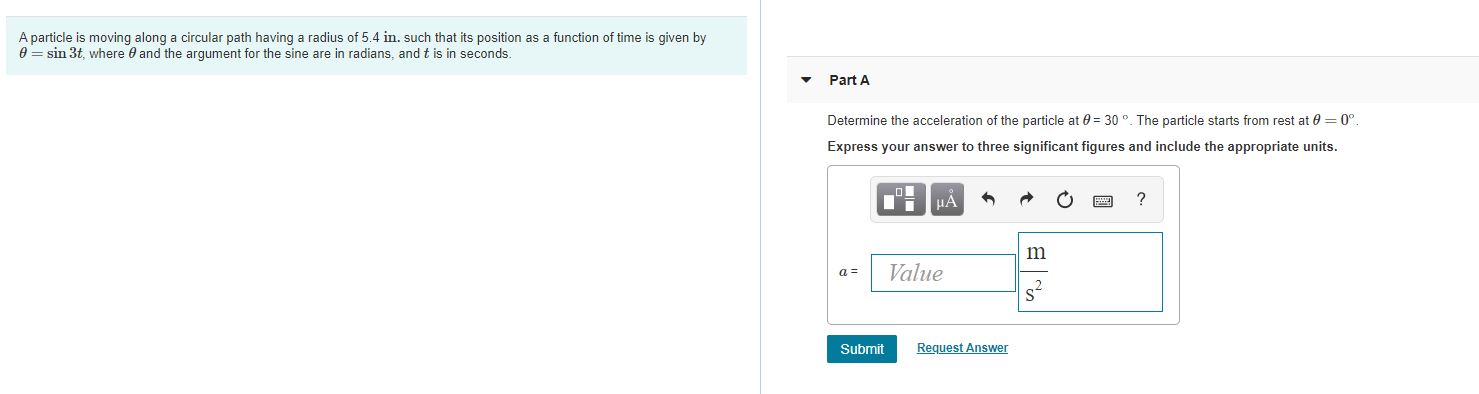A particle is moving along a circular path having a radius of 5.4 in. such that its position as a function of time is given by @=sin 3t, where and the argument for the sine are in radians, and t is in seconds. Part A Determine the acceleration of the particle at 8 = 30. The particle starts from rest at 0 = 0° Express your answer to three significant figures and include the appropriate units. Value Submit Request Answer

• ### A freight train travels at v= 54 (1 – e-t) ft/s, where t is the elapsed...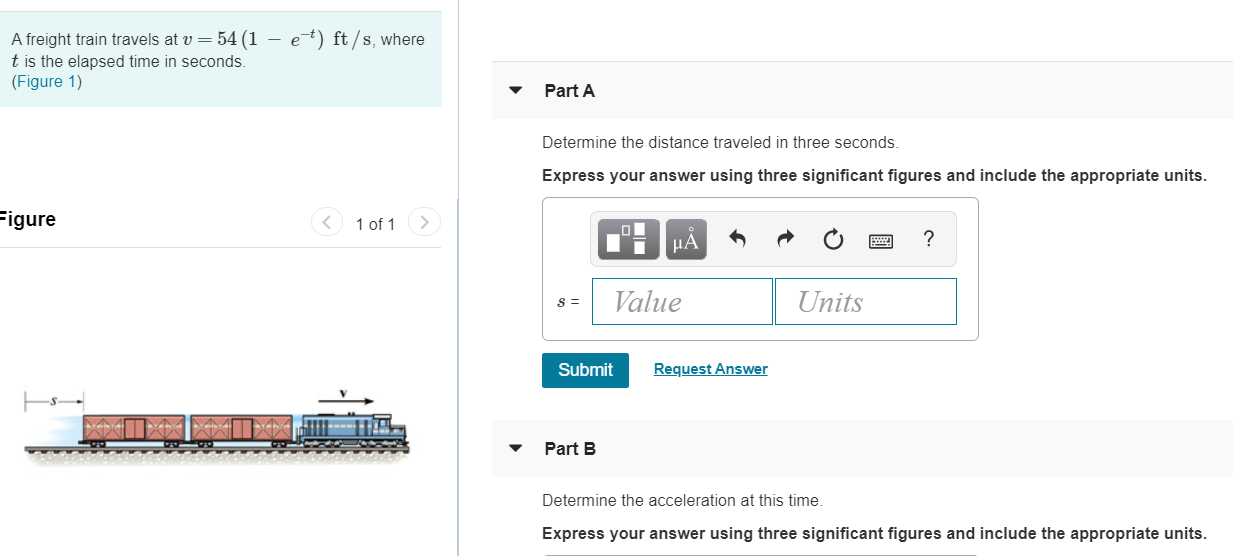A freight train travels at v= 54 (1 – e-t) ft/s, where t is the elapsed time in seconds. (Figure 1) Part A Determine the distance traveled in three seconds Express your answer using three significant figures and include the appropriate units. Figure (< 1 of 1 > : HÅR ? O 2 Units 8 = Value Submit Request Answer Part B Determine the acceleration at this time Express your answer using three significant figures and include the appropriate units.

• ### A boat has an initial speed of 19 ft/s·It then increases its speed along a orcular...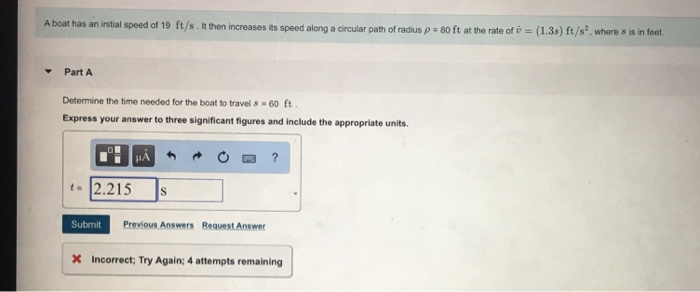A boat has an initial speed of 19 ft/s·It then increases its speed along a orcular path of radius 80 ft at the rate of U-(1.3s) ft/s2, where s is in feet. Part A Determine the time needed for the boat to travel 8-60 ft Express your answer to three significant figures and include the appropriate units t 2.215 S Submit Previous Answers Request Answer X Incorrect: Try Again; 4 attempts remaining

• ### To calculate the normal and tangential components of the acceleration of an object along a given...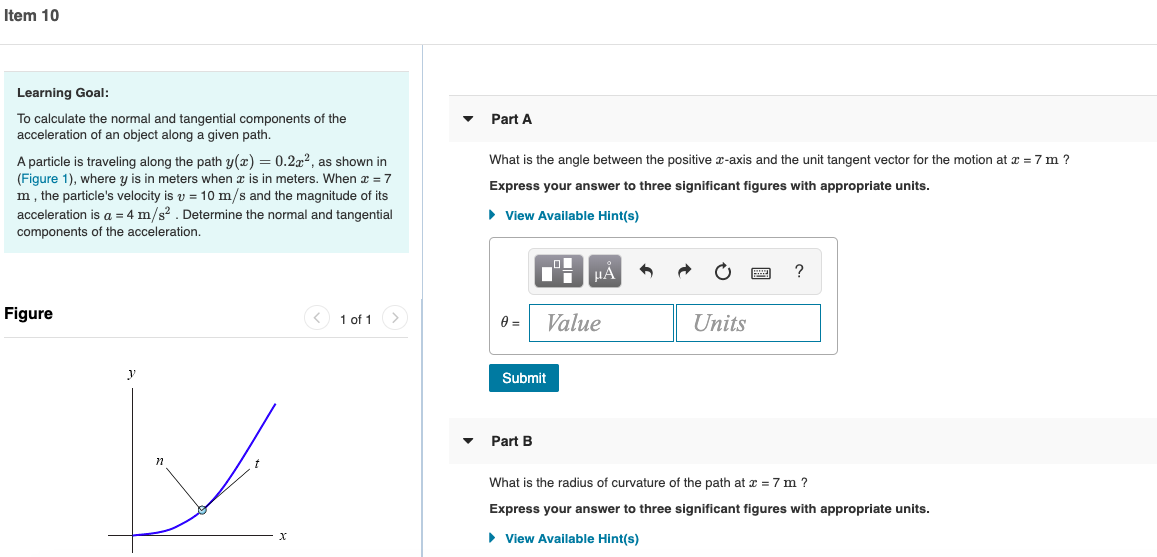To calculate the normal and tangential components of the acceleration of an object along a given path. A particle is traveling along the path y(x)=0.2x2y(x)=0.2x2, as shown in (Figure 1), where yy is in meters when xx is in meters. When xxx = 7 mm , the particle's velocity is vvv = 10 m/sm/s and the magnitude of its acceleration is aaam = 4 m/s2m/s2 . Determine the normal and tangential components of the acceleration. Item 10 Learning Goal: To...

• ### tem 19 19 of 20 A particle is moving along a circular path having a radius...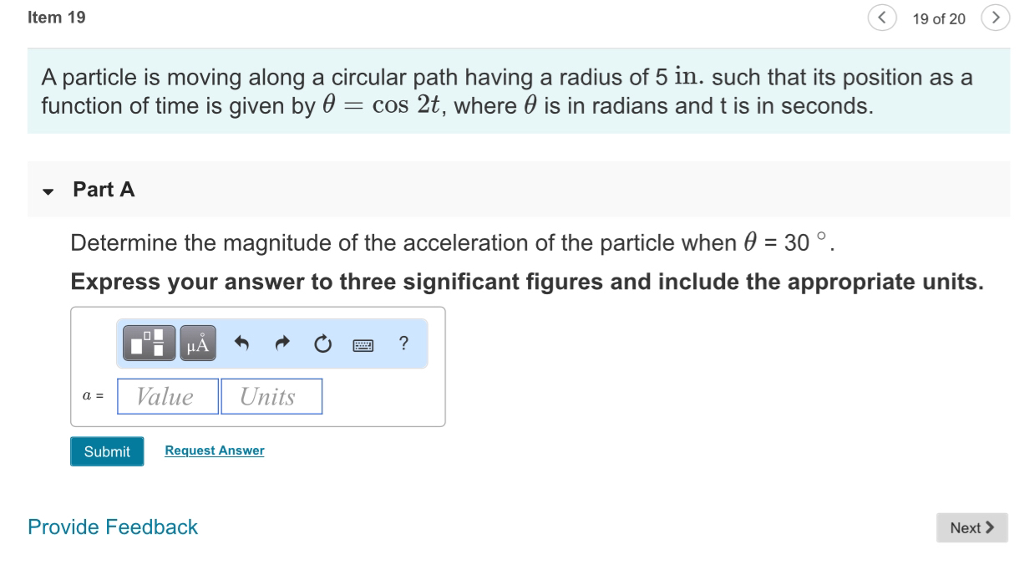tem 19 19 of 20 A particle is moving along a circular path having a radius of 5 in. such that its position as a function of time is given by θ-cos 2t, where θ is in radians and t is in seconds. - Part A 。. Determine the magnitude of the acceleration of the particle when 30 Express your answer to three significant figures and include the appropriate units. 0図? ValueUnits Submit Request Answer Provide Feedback Next >